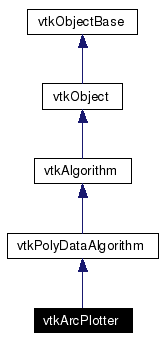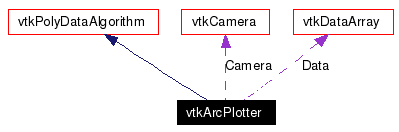# vtkArcPlotter Class Reference

`#include <vtkArcPlotter.h>`

Inheritance diagram for vtkArcPlotter:[legend]
Collaboration diagram for vtkArcPlotter:[legend]
List of all members.

## Detailed Description

plot data along an arbitrary polyline

vtkArcPlotter performs plotting of attribute data along polylines defined with an input vtkPolyData data object. Any type of attribute data can be plotted including scalars, vectors, tensors, normals, texture coordinates, and field data. Either one or multiple data components can be plotted.

To use this class you must specify an input data set that contains one or more polylines, and some attribute data including which component of the attribute data. (By default, this class processes the first component of scalar data.) You will also need to set an offset radius (the distance of the polyline to the median line of the plot), a width for the plot (the distance that the minimum and maximum plot values are mapped into), an possibly an offset (used to offset attribute data with multiple components).

Normally the filter automatically computes normals for generating the offset arc plot. However, you can specify a default normal and use that instead.

See also:
vtkXYPlotActor
Tests:
vtkArcPlotter (Tests)

Definition at line 60 of file vtkArcPlotter.h.

## Public Types

typedef vtkPolyDataAlgorithm Superclass

## Public Member Functions

virtual const char * GetClassName ()
virtual int IsA (const char *type)
unsigned long GetMTime ()
void PrintSelf (ostream &os, vtkIndent indent)
virtual void SetCamera (vtkCamera *)
virtual vtkCameraGetCamera ()
virtual void SetPlotMode (int)
virtual int GetPlotMode ()
void SetPlotModeToPlotScalars ()
void SetPlotModeToPlotVectors ()
void SetPlotModeToPlotNormals ()
void SetPlotModeToPlotTCoords ()
void SetPlotModeToPlotTensors ()
void SetPlotModeToPlotFieldData ()
virtual void SetPlotComponent (int)
virtual int GetPlotComponent ()
virtual void SetRadius (double)
virtual double GetRadius ()
virtual void SetHeight (double)
virtual double GetHeight ()
virtual void SetOffset (double)
virtual double GetOffset ()
virtual void SetUseDefaultNormal (int)
virtual int GetUseDefaultNormal ()
virtual void UseDefaultNormalOn ()
virtual void UseDefaultNormalOff ()
virtual void SetDefaultNormal (float, float, float)
virtual void SetDefaultNormal (float)
virtual float * GetDefaultNormal ()
virtual void GetDefaultNormal (float data)
virtual void SetFieldDataArray (int)
virtual int GetFieldDataArray ()

## Static Public Member Functions

static vtkArcPlotterNew ()
static int IsTypeOf (const char *type)
static vtkArcPlotterSafeDownCast (vtkObject *o)

## Protected Member Functions

vtkArcPlotter ()
~vtkArcPlotter ()
int RequestData (vtkInformation *, vtkInformationVector **, vtkInformationVector *)
int OffsetPoint (vtkIdType ptId, vtkPoints *inPts, double n, vtkPoints *newPts, double offset, double *range, double val)
int ProcessComponents (vtkIdType numPts, vtkPointData *pd)

## Protected Attributes

vtkCameraCamera
int PlotMode
int PlotComponent
double Radius
double Height
double Offset
float DefaultNormal 
int UseDefaultNormal
int FieldDataArray

## Member Typedef Documentation

 Reimplemented from vtkPolyDataAlgorithm. Definition at line 66 of file vtkArcPlotter.h.

## Constructor & Destructor Documentation

 vtkArcPlotter::vtkArcPlotter ( ) ` [protected]`

 vtkArcPlotter::~vtkArcPlotter ( ) ` [protected]`

## Member Function Documentation

 static vtkArcPlotter* vtkArcPlotter::New ( ) ` [static]`
 Instantiate with no default camera and plot mode set to VTK_SCALARS. Reimplemented from vtkPolyDataAlgorithm.

 virtual const char* vtkArcPlotter::GetClassName ( ) ` [virtual]`
 Reimplemented from vtkPolyDataAlgorithm.

 static int vtkArcPlotter::IsTypeOf ( const char * type ) ` [static]`
 Return 1 if this class type is the same type of (or a subclass of) the named class. Returns 0 otherwise. This method works in combination with vtkTypeRevisionMacro found in vtkSetGet.h. Reimplemented from vtkPolyDataAlgorithm.

 virtual int vtkArcPlotter::IsA ( const char * type ) ` [virtual]`
 Return 1 if this class is the same type of (or a subclass of) the named class. Returns 0 otherwise. This method works in combination with vtkTypeRevisionMacro found in vtkSetGet.h. Reimplemented from vtkPolyDataAlgorithm.

 static vtkArcPlotter* vtkArcPlotter::SafeDownCast ( vtkObject * o ) ` [static]`
 Reimplemented from vtkPolyDataAlgorithm.

 void vtkArcPlotter::PrintSelf ( ostream & os, vtkIndent indent ) ` [virtual]`
 Methods invoked by print to print information about the object including superclasses. Typically not called by the user (use Print() instead) but used in the hierarchical print process to combine the output of several classes. Reimplemented from vtkPolyDataAlgorithm.

 virtual void vtkArcPlotter::SetCamera ( vtkCamera * ) ` [virtual]`
 Specify a camera used to orient the plot along the arc. If no camera is specified, then the orientation of the plot is arbitrary.

 virtual vtkCamera* vtkArcPlotter::GetCamera ( ) ` [virtual]`

 virtual void vtkArcPlotter::SetPlotMode ( int ) ` [virtual]`
 Specify which data to plot: scalars, vectors, normals, texture coords, tensors, or field data. If the data has more than one component, use the method SetPlotComponent to control which component to plot.

 virtual int vtkArcPlotter::GetPlotMode ( ) ` [virtual]`
 Specify which data to plot: scalars, vectors, normals, texture coords, tensors, or field data. If the data has more than one component, use the method SetPlotComponent to control which component to plot.

 void vtkArcPlotter::SetPlotModeToPlotScalars ( ) ` [inline]`
 Specify which data to plot: scalars, vectors, normals, texture coords, tensors, or field data. If the data has more than one component, use the method SetPlotComponent to control which component to plot. Definition at line 83 of file vtkArcPlotter.h. References VTK_PLOT_SCALARS.

 void vtkArcPlotter::SetPlotModeToPlotVectors ( ) ` [inline]`
 Specify which data to plot: scalars, vectors, normals, texture coords, tensors, or field data. If the data has more than one component, use the method SetPlotComponent to control which component to plot. Definition at line 84 of file vtkArcPlotter.h. References VTK_PLOT_VECTORS.

 void vtkArcPlotter::SetPlotModeToPlotNormals ( ) ` [inline]`
 Specify which data to plot: scalars, vectors, normals, texture coords, tensors, or field data. If the data has more than one component, use the method SetPlotComponent to control which component to plot. Definition at line 85 of file vtkArcPlotter.h. References VTK_PLOT_NORMALS.

 void vtkArcPlotter::SetPlotModeToPlotTCoords ( ) ` [inline]`
 Specify which data to plot: scalars, vectors, normals, texture coords, tensors, or field data. If the data has more than one component, use the method SetPlotComponent to control which component to plot. Definition at line 86 of file vtkArcPlotter.h. References VTK_PLOT_TCOORDS.

 void vtkArcPlotter::SetPlotModeToPlotTensors ( ) ` [inline]`
 Specify which data to plot: scalars, vectors, normals, texture coords, tensors, or field data. If the data has more than one component, use the method SetPlotComponent to control which component to plot. Definition at line 87 of file vtkArcPlotter.h. References VTK_PLOT_TENSORS.

 void vtkArcPlotter::SetPlotModeToPlotFieldData ( ) ` [inline]`
 Specify which data to plot: scalars, vectors, normals, texture coords, tensors, or field data. If the data has more than one component, use the method SetPlotComponent to control which component to plot. Definition at line 88 of file vtkArcPlotter.h. References VTK_PLOT_FIELD_DATA.

 virtual void vtkArcPlotter::SetPlotComponent ( int ) ` [virtual]`
 Set/Get the component number to plot if the data has more than one component. If the value of the plot component is == (-1), then all the components will be plotted.

 virtual int vtkArcPlotter::GetPlotComponent ( ) ` [virtual]`
 Set/Get the component number to plot if the data has more than one component. If the value of the plot component is == (-1), then all the components will be plotted.

 virtual void vtkArcPlotter::SetRadius ( double ) ` [virtual]`
 Set the radius of the "median" value of the first plotted component.

 virtual double vtkArcPlotter::GetRadius ( ) ` [virtual]`
 Set the radius of the "median" value of the first plotted component.

 virtual void vtkArcPlotter::SetHeight ( double ) ` [virtual]`
 Set the height of the plot. (The radius combined with the height define the location of the plot relative to the generating polyline.)

 virtual double vtkArcPlotter::GetHeight ( ) ` [virtual]`
 Set the height of the plot. (The radius combined with the height define the location of the plot relative to the generating polyline.)

 virtual void vtkArcPlotter::SetOffset ( double ) ` [virtual]`
 Specify an offset that translates each subsequent plot (if there is more than one component plotted) from the defining arc (i.e., polyline).

 virtual double vtkArcPlotter::GetOffset ( ) ` [virtual]`
 Specify an offset that translates each subsequent plot (if there is more than one component plotted) from the defining arc (i.e., polyline).

 virtual void vtkArcPlotter::SetUseDefaultNormal ( int ) ` [virtual]`
 Set a boolean to control whether to use default normals. By default, normals are automatically computed from the generating polyline and camera.

 virtual int vtkArcPlotter::GetUseDefaultNormal ( ) ` [virtual]`
 Set a boolean to control whether to use default normals. By default, normals are automatically computed from the generating polyline and camera.

 virtual void vtkArcPlotter::UseDefaultNormalOn ( ) ` [virtual]`
 Set a boolean to control whether to use default normals. By default, normals are automatically computed from the generating polyline and camera.

 virtual void vtkArcPlotter::UseDefaultNormalOff ( ) ` [virtual]`
 Set a boolean to control whether to use default normals. By default, normals are automatically computed from the generating polyline and camera.

 virtual void vtkArcPlotter::SetDefaultNormal ( float , float , float ) ` [virtual]`
 Set the default normal to use if you do not wish automatic normal calculation. The arc plot will be generated using this normal.

 virtual void vtkArcPlotter::SetDefaultNormal ( float  ) ` [virtual]`
 Set the default normal to use if you do not wish automatic normal calculation. The arc plot will be generated using this normal.

 virtual float* vtkArcPlotter::GetDefaultNormal ( ) ` [virtual]`
 Set the default normal to use if you do not wish automatic normal calculation. The arc plot will be generated using this normal.

 virtual void vtkArcPlotter::GetDefaultNormal ( float data ) ` [virtual]`
 Set the default normal to use if you do not wish automatic normal calculation. The arc plot will be generated using this normal.

 virtual void vtkArcPlotter::SetFieldDataArray ( int ) ` [virtual]`
 Set/Get the field data array to plot. This instance variable is only applicable if field data is plotted.

 virtual int vtkArcPlotter::GetFieldDataArray ( ) ` [virtual]`
 Set/Get the field data array to plot. This instance variable is only applicable if field data is plotted.

 unsigned long vtkArcPlotter::GetMTime ( ) ` [virtual]`
 New GetMTime because of camera dependency. Reimplemented from vtkObject.

 int vtkArcPlotter::RequestData ( vtkInformation * , vtkInformationVector ** , vtkInformationVector * ) ` [protected, virtual]`
 This is called by the superclass. This is the method you should override. Reimplemented from vtkPolyDataAlgorithm.

 int vtkArcPlotter::OffsetPoint ( vtkIdType ptId, vtkPoints * inPts, double n, vtkPoints * newPts, double offset, double * range, double val ) ` [protected]`

 int vtkArcPlotter::ProcessComponents ( vtkIdType numPts, vtkPointData * pd ) ` [protected]`

## Member Data Documentation

 vtkCamera* vtkArcPlotter::Camera` [protected]`
 Definition at line 157 of file vtkArcPlotter.h.

 int vtkArcPlotter::PlotMode` [protected]`
 Definition at line 158 of file vtkArcPlotter.h.

 int vtkArcPlotter::PlotComponent` [protected]`
 Definition at line 159 of file vtkArcPlotter.h.

 double vtkArcPlotter::Radius` [protected]`
 Definition at line 160 of file vtkArcPlotter.h.

 double vtkArcPlotter::Height` [protected]`
 Definition at line 161 of file vtkArcPlotter.h.

 double vtkArcPlotter::Offset` [protected]`
 Definition at line 162 of file vtkArcPlotter.h.

 float vtkArcPlotter::DefaultNormal` [protected]`
 Definition at line 163 of file vtkArcPlotter.h.

 int vtkArcPlotter::UseDefaultNormal` [protected]`
 Definition at line 164 of file vtkArcPlotter.h.

 int vtkArcPlotter::FieldDataArray` [protected]`
 Definition at line 165 of file vtkArcPlotter.h.

The documentation for this class was generated from the following file:

Generated on Mon Jan 21 23:33:57 2008 for VTK by1.4.3-20050530